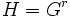# Isomorphic nth powers not implies isomorphic

Suppose$n > 1$. Then, we can find groups$G, H$ such that$G^n \cong H^n$ (i..e, the$n^{th}$ direct powers are isomorphic groups) but$G \not \cong H$. In fact, we can choose both$G$ and$H$ to be countable torsion-free (i.e., aperiodic) abelian groups.
1. There exist abelian groups whose isomorphism classes of direct powers have any given period: This states that for any$r$, we can find a (countable torsion-free) abelian group$G$ such that$G^a \cong G^b \iff a \equiv b \pmod r$.
Pick$r = n$ from fact (1), let$G$ be the group$G$ as arising from fact (1), and let$H = G^r$. Then,$H^r = (G^r)^r = G^{r^2}$ Since$r^2 \equiv r \pmod r$, we obtain that$H^r \cong G^r$. However, since$r \not \equiv 1 \pmod r$,$H \not \cong G$. This gives the required example.